# AN EXAMINATION OF THE MOTION OF AN OBLATE INFINITESIMAL BODY IN THE GENERALIZED PHOTOGRAVITATIONAL ER3BP WITH CIRCUMBINARY DISC

• 0 Review(s)

Product Category: Projects

Product Code: 00006564

No of Pages: 117

No of Chapters: 1-5

File Format: Microsoft Word

Price :

\$20

ABSTRACT

This study examined the motion of an oblate infinitesimal body under the influence of two finite luminous bodies together with the gravitational potential from a disc, within the framework of the elliptic restricted three body problem. The locations and stability of the equilibrium points with respect to the eccentricity, oblateness of the third body, radiation pressure parameters and gravitational potential from a disc were established. A close examination of mc, shows that all the parameters cause a reduction in the size of the region of stability confirming their destabilizing tendencies, while the gravitational potential from the circumbinary disc has a stabilizing effect. The study also, numerically highlights the influence of the aforementioned parameters on the locations/stability of the triangular and collinear points for the binary systems HD98800B and GJ630.1A. It is observed that the triangular points are stable for 0 < m < mc  and unstable for m< m < 1/and in the case of collinear points we see that the equation determining the distance ratio among the three masses is an eleventh-order polynomial. The collinear points however remain unstable even under the effects of radiation pressure of both the primaries, oblateness of the third body and potential from a disc.

Declaration iii
Certification i
Acknowledgement v
Dedication vii
Abstract vi
List of Tables xiii
List of Figures xv
List of Symbols xvii

CHAPTER ONE
INTRODUCTION
1.1 Background of the Study 1
1.2 Statement of the Problem 4
1.3 Aim and Objectives 5
1.4 Significance of the Study 6
1.5 Justification of the Study 6
1.6 Research Methodology 6
1.7 Theoretical Frame Work 7
1.7.1 Kepler's Laws of Planetary Motion 7
1.7.2 Three-Body Problem 8
1.7.4 Oblateness 9
1.7.5 Eccentricity 10
1.7.6 Synodic or Rotating Co-ordinate System 10
1.7.7 Equilibrium Point and Stability of Equilibrium Point 10
1.7.8 Stability of a Linear System 11
1.7.9 Binary Star System 11
1.7.10 Circumbinary Planets 12
1.7.11 Circumbinary Disc 12
1.7.12 Protoplanetary Disc 13
1.7.13 Debris Disc 13
1.7.14 Asteroid belt 14
1.7.15 Asteroid with Disc 14
1.7.16 CM Draconis 15
1.7.17 HD 98800 16
1.7.18 Exoplanets 17

CHAPTER TWO
LITERATURE REVIEW
2.1 Introduction 18
2.2 The Restricted Three-Body Problem 18
2.3 The Elliptic Restricted Three-Body Problem 19
2.4 Related Literatures 20

CHAPTER THREE
LOCATIONS AND LINEAR STABILITY OF EQUILIBRIUM POINTS
3.1 Introduction 25
3.2 Equations of Motion 25
3.3 Variational Equation 26
3.4 Characteristic Equation 27
3.5 Jacobian Integral 28
3.6 Locations of the Equilibrium Points 29
3.6.1 Locations of triangular equilibrium points 29
3.6.2 Locations of the Collinear Equilibrium Points 33
3.7 Linear Stability of the equilibrium points 37
3.7.1 Stability of the Triangular Equilibrium Points 40
3.7.2 Stability of the Collinear Equilibrium Points 46

CHAPTER FOUR
NUMERICAL COMPUTATIONS
4.1 Triangular Equilibrium Points 51
4.2 Stability of triangular equilibrium points 61
4.3 Numerical Results of the Collinear Equilibrium Points 64
4.4 Diagrams of numerical Results of the Collinear Equilibrium Points of the models 66
4.5 Discussion 82
The critical mass value 82

CHAPTER FIVE
SUMMARY, CONCLUSION AND RECOMMENDATIONS
5.1 Summary 84
5.2 Conclusion 84
5.3 Recommendations 86
5.4 Future Research 86
5.5 Contribution to Knowledge 87
REFERENCES 88
APPENDICES 95
Appendix 1: Triangular Points 95
Appendix 2: Stability Region 96
Appendix 3: Collinear Points 98

LIST OF TABLES

Table 4. 1: Relevant Numerical Data 51
Table 4. 2: Locations and Stability of Triangular Equilibrium Points 52
Table 4. 3: Effect of eccentricity on L4,5 in the binary system HD98800B 53
Table 4. 4: Effect of eccentricity on L4,5 in the binary system GJ 630.1A 54
Table 4. 5: Effect of semi-major axis on L4,5 in the binary system HD98800B 55
Table 4. 6: Effect of semi-major axis on L4,5 in the binary system GJ 630.1A 56
Table 4. 7: Effect of oblateness of the third body on L4,5 in the binary system HD98800B 57
Table 4. 8: Effect of oblateness of the third body on L4,5 in the binary system GJ 630.1A 58
Table 4. 9: Effect of potential from the disc on L4,5 in the binary system HD98800B 59
Table 4. 10: Effect of potential from the disc on L4,5 in the binary system GJ 630.1A 60
Table 4. 11: Locations of collinear points for m = 0.453125, q1 = 0.99903, q2 = 0.99941, A = Mb  = T = 0.01
Table 4. 12: Effect of eccentricity in HD 98800 B on the collinear point L1 66
Table 4. 13: Effect of eccentricity in GJ 630.1A on the collinear point L1 67
Table 4. 14: Effect of semi major-axis in HD 98800 B on the collinear point L1 67
Table 4. 15: Effect of semi major-axis in GJ 630.1A on the collinear point L1 68
Table 4. 16: Effect of oblateness of third body in HD 98800 B on the collinear point L1 68
Table 4. 17: Effect of oblateness of third body in GJ 630.1A on the collinear point L1 69
Table 4. 18: Effect of potential from the disc in HD 98800 B on the collinear point L1 69
Table 4. 19: Effect of potential from the disc in GJ 630 1.A on the collinear point L1 70
Table 4. 20: Effect of eccentricity in HD 98800 B on the collinear point L2 70
Table 4. 21: Effect of eccentricity in GJ 630.1A on the collinear point L2 71
Table 4. 22: Effect of semi major-axis in HD 98800 B on the collinear point L2 71
Table 4. 23: Effect of semi major-axis in GJ 630.1A on the collinear point L2 72
Table 4. 24: Effect of oblateness of third body in HD 98800 B on the collinear point L2 72
Table 4. 25: Effect of oblateness of third body in GJ 630 1.A on the collinear point L2 73
Table 4. 26: Effect of potential from the disc in HD98800B on the collinear points L2 73
Table 4. 27: Effect of potential from the disc in GJ 630 1.A on the collinear point L2 74
Table 4. 28: Effect of eccentricity in HD 98800 B on the collinear point L3 74
Table 4. 29: Effect of eccentricity in GJ 630.1A on the collinear point L3 75
Table 4. 30: Effect of semi major-axis in HD 98800 B on the collinear point L3 75
Table 4. 31: Effect of semi major-axis in GJ 630.1A on the collinear point L3 76
Table 4. 32: Effect of oblateness of third body in HD 98800 B on the collinear point L3 76
Table 4. 33: Effect of oblateness of third body in GJ630 1.A on the collinear point L3 77
Table 4. 34: Effect of potential from the disc in HD 98800 B on the collinear point L3 77
Table 4. 35: Effect of potential from the disc in GJ 630 1.A on the collinear points L3 78

LIST OF FIGURES

Figure 1. 1: The shape of an oblate spheroid 9

Figure 1. 2: Artists impression of HD 98800. ( a model under consideration on page 57 ) 16

Figure 3. 1: Position of the collinear equilibrium point L1 35

Figure 3. 2: Position of the collinear equilibrium point L2 35

Figure 3. 3: Position of the collinear equilibrium point L3 36

Figure 4. 1: Effect of eccentricity on L4,5 in the binary system HD98800B 54

Figure 4. 2: Effect of eccentricity on L4,5 in the binary system GJ 630.1A 55

Figure 4. 3: Effect of semi-major axis on L4,5 in the binary system HD98800B 56

Figure 4. 4: Effect of semi-major axis on L4,5 in the binary system GJ 630.1A 57

Figure 4. 5: Effect of oblateness of the third body on L4,5 in the binary system HD98800B 58

Figure 4. 6: Effect of oblateness of the third body on L4,5 in the binary system GJ 630.1A 59

Figure 4. 7: Effect of potential from the disc on L4,5 in the binary system HD98800B 60

Figure 4. 8: Effect of potential from the disc on L4,5 in the binary system GJ 630.1A 61

Figure 4. 9: Effect of semi-major axis on the stability L4,5 in HD 98800 B 61

Figure 4. 10: Effect of semi-major axis on the stability L4,5 in GJ 630.1A 62

Figure 4. 11: Effect of oblateness on the stability L4,5 in HD 98800 B 62

Figure 4. 12: Effect of oblateness on the stability L4,5 in GJ 630.1A 63

Figure 4. 13: Effect of potential from the disc on the stability L4,5 in HD98800B 63

Figure 4. 14: Effect of potential from the disc on the stability L4,5 in GJ 630.1A 64

Figure 4. 15: Effect of eccentricity on the collinear points Li (i=1,2,3) of HD98800 B 78

Figure 4. 16: Effect of eccentricity on the collinear points Li (i=1,2,3) of GJ 630.1A 79

Figure 4. 17:Effect of semi-major axis on the collinear points Li (i=1,2,3) of HD98800 B 79

Figure 4. 18: Effect of semi-major axis on the collinear points Li (i=1,2,3) of GJ 630.1A 80

Figure 4. 19: Effect of third body on the collinear points Li (i=1,2,3) of HD 98800 B 80

Figure 4. 20: Effect of third body on the collinear points Li (i=1,2,3) of GJ 630.1A 81

Figure 4. 21: Effect of potential from the disc on the collinear points Li (i=1,2,3) of HD98800 B 81

Figure 4. 22: Effect of potential from the disc on the collinear points Li (i=1,2,3) of 82

LIST OF SYMBOLS

x ,and z   Are the Coordinates
m Mass ratio
mc Critical value of the mass parameter
m1 Mass of the bigger primary
m2 Mass of the smaller primary
m3 Mass of the infinitesimal body
W Potential force function
A Oblateness of the infinitesimal body
r1 Distance from the third body to the bigger primary
r2 Distance from the third body to the smaller primary
rc Radial distance of the infinitesimal body
Mb Disc potential
T Determines the density profile of the circular cluster of material points
a = 1 - a  Semi-major axis of the system, where  is a small value less than 1 (i.e. 0 < a << 1  )
e Eccentricity of the orbits of the primaries
qi = 1 - bi Radiation coefficient, where is the ratio of the radiation pressure force to the gravitational force of the primaries, bi << 1, ( i = 1,2 )
n Mean motion

CHAPTER ONE
INTRODUCTION

1.1 Background of the Study
In a three-body problem, three bodies move in space under their mutual gravitational interactions as described by Newton's law of gravitation. Solutions of this problem require that future and past motions of the bodies be determined uniquely based solely on their present positions and velocities. In general, the motions of the bodies take place in three dimensions (3D), and there are no restrictions on their masses or on the initial conditions. Thus, this is referred to as the general three-body problem. At first glance, the difficulty of the problem is not obvious, especially when considering that the two-body problem has well-known closed form solutions given in terms of elementary functions.

Adding one extra body makes the problem too complicated to obtain similar types of solutions. In the past, many physicists, astronomers and mathematicians attempted unsuccessfully to find closed form solutions to the three-body problem. Such solutions do not exist because motions of the three bodies are in general unpredictable, which makes the three-body problem one of the most challenging problems in the history of science.

In celestial mechanics, the general three-body problem deals with gravitationally interacting astronomical bodies and intends to predict their motions. In our Solar System, the planets and asteroids move around the Sun, while the moons orbit their host planets, which in turn also move around the Sun. As typical examples of the three-body problem, consider the Sun-Planet-Planet, Sun-Planet-Moon, or Sun-Planet- Asteroid systems. The three-body problem representing the latter system can be significantly simplified because the mass of the asteroid is always negligible when compared to the mass of either the Sun or the Planet, which means that the gravitational influence of the Asteroid on the Planet and the Sun can be omitted from the theory, If this condition is satisfied, then the general three-body problem becomes the restricted three-body problem, and there are two possibilities, namely, the two bodies with dominant masses move around their center of mass either along circular or elliptic orbits, which leads to the respective circular or elliptic restricted three-body problems. It is the circular restricted three-body problem (CR3BP) that has been the most extensively studied. The three-body problem has been studied for over three hundred years.

A brief historical overview of the most significant results that have contributed to the current developments in the 3BP can be divide into two parts: first, the time period from the publication of Newton's Principia in 1687, to the publication of Poincare's Les M'ethodes Nouvelles de la M'ecanique C'eleste published in 1892(volume 1 and 2) and in 1899 (volume3).

The three-body problem was formulated and studied by Newton in 1687 in his Principia, where he considered the motion of the Earth and the Moon around the Sun. In this problem, there are key elements such as the ratio of the Moon's mass, MM, to the Earth's mass, ME, that is MM/ME = 0.0123, which is a small but not negligible number. Another element is the tilt of the Moon's orbit to the Earth's orbit, about 5°. This makes the problem completely predictable despite the fact that the Earth's orbit around the Sun and the Moon's orbit around the Earth are almost perfect circles. Newton stated that the problem was very difficult to solve; however, he was able to obtain an approximate solution which agreed with observations to within 8%.

A special form of the general three-body problem was proposed by Euler in 1767. He considered three bodies of arbitrary (finite) masses and placed them along a straight line. Euler showed that the bodies would always stay on this line for suitable initial conditions, and that the line would rotate about the center of mass of the bodies, leading to periodic motions of all three bodies along ellipses. Around the same time, Lagrange in 1772 found a second class of periodic orbits in the general three-body problem. He showed that if the bodies were positioned in such a way that they form a triangle of equal sides which would move along ellipses for certain initial conditions, preserving always their original configuration. The Euler and Lagrange solutions are now known as particular solutions to the general three-body problem.

Euler in 1767 was the first to formulate the CR3BP in a rotating (or synodic) coordinate system. This was an important development in the study of the three-body problem. Lagrange also studied the CR3BP and demonstrated that there were five equilibrium points (now known as the Lagrange points) at which the gravitational forces of the bodies balanced out; the Trojan asteroids discovered in 1906 along Jupiter's orbit occupy space close to two of the Lagrange points. Important contributions to the CR3BP were made by Jacobi in 1836; he used the synodic (rotating) coordinate system originally introduced by Euler in 1767 to demonstrate that there was an integral of motion, which is now named after him. The Jacobi integral was used by Hill in 1877and 1878 to determine the motion of an asteroid in the three-body problem and to introduce the so-called zero velocity curves (ZVC), which establish regions in space where the bodies are allowed to move. Hill considered a special case of the CR3BP in which two masses were much smaller than the first one (the problem is now known as the Hill problem), and in this way, he discovered a new class of periodic solutions. His main contribution was to present a new approach to solve the Sun-Earth-Moon three-body problem, After almost two hundred years since the original formulation of the problem by Newton 1687, Hill developed his lunar theory, with some modifications made by Brown in 1896.

In the second half of the nineteenth century, Poincare studied and advanced the solution of the Three-body problem. His monumental three-volume book Les M'ethodes Nouvelles de la M'ecanique C'eleste, originally published in 1892-99, contains his most important contribution to the study of the CR3BP; the book was translated as New Methods of Celestial Mechanics and edited by L. D. Goroff in 1993. In this work, Poincare developed a number of new qualitative methods to solve differential equations and used them to identify and study possible periodic orbits, while demonstrating the non integrability of the system of equations describing the three-body problem. Poincare's new methods allowed him to identify the unpredictability of the problem, and to discover the first manifestation of a new phenomenon, which is now commonly known as chaos, Poincare submitted some of those results for the King Oscar II Birthday Competition and he was awarded the prize ( Musielak & Quarles, 2015).

1.2 Statement of the Problem

The orbits of most celestial bodies are elliptic, which has prompted many researches (Kumar and Choudry, 1990; Markellos et al., 1992; Zimovschikov and Tkhai, 2004; Ammar, 2008; Kumar and Ishwar, 2009, 2011). Recently, Singh and Umar (2012) have investigated the motion of a dust particle, in an orbit with dark oblate, degenerate primary and a stellar secondary companion; around triangular equilibrium points when both oblate and luminous primaries move around their common center of mass respectively, in the framework of the ER3BP.

There are ring-type discs of dust particles in the stellar systems which are regarded as young analogues of the Kuiper belt (Singh and Taura, 2014). Of an observed 69 A3-F8 main sequence binary star systems, nearly 60% showed dust rings surrounding binary stars. This inspired many scientists to study the CR3BP by taking into consideration the additional gravitational potential from the disc (Pitjeva & Pitjev 2014, Kushvah (2008), Singh & Taura (2013, 2014a, 2014b), lorio (2007, 2012)). In their work Yeh and Jiang (2006) have included the gravitational potential from the belt in their studies of the CR3BP. Also, Singh and Taura (2013) examined the combined effect of radiation and oblateness of both primaries, together with additional gravitational potential from the disc on the motion of an infinitesimal body in the CR3BP. They, Singh and Taura (2013) studied the effects of oblateness up to J4 of the less massive primary and gravitational potential from a belt on the linear stability of triangular equilibrium points in the photogravitational CR3BP, They found that the gravitational potential from the disc makes the structure of the CR3BP quite different such that new libration points exist under certain conditions. Also, the orbital motion of a test particle around a primary is greatly affected in the presence of a massive disc (lorio, 2007).

Hence this research examines the dynamics of an oblate circumbinary planet in the vicinity of luminous primaries moving in elliptic orbits about their common barycenter, surrounded by ring-type discs. Thus, apart from the Newtonian gravitational attraction between the primaries, the effects of the luminosity of both primaries, oblateness of the circumbinary planet and eccentricity of the orbits of the primaries, an additional gravitational potential from the disc has been considered in this study.

1.3 Aim and Objectives

The aim of this research is to determine the motion of an oblate infinitesimal body in the generalized photogravitational ER3BP with a circumbinary disc when both primaries are sources of radiation. This is  achieved through the following objectives:

i. Determining the effect of the parameters on the locations and stability of the triangular equilibrium points

ii. Determining the effect of the parameters on the locations and stability of collinear equilibrium points

iii. Applying the results to obtain numerically the triangular points and determine the stability of the oblate circumbinary planet in the neighborhood of HD 98800 B and GJ630.1A

1.4 Significance of the Study

In recent studies, many perturbing forces such as radiation, variation of the masses, Poynting-Robertson drag (P-R drag), oblateness and triaxiality, have been included in the study of the circular or elliptic R3BP.The orbits of the celestial bodies are mostly elliptic not circular. It is therefore more accurate to study the ER3BP, because it has a significant effect which the CR3BP neglects.

1.5 Justification of the Study

The human civilization is no longer bound to its environment via land and water, but has evolved to a space-faring civilization. So the expansion into space, in terms of artificial satellites, requires the formulation of new models that include the effects of some of the perturbing forces on the satellite. Motion in the elliptic restricted three-body problem is one of such models which consider the effects of radiation of the primaries, an oblate infinitesimal body and gravitational potential from a disc. Thus, this model will be very helpful in the study of binary stars.

1.6 Research Methodology

Following the work of Singh and Umar (2012), in which they studied the ER3BP under oblate and radiating primaries in barycentric, pulsating and non-dimensional co-ordinates, and the work of Singh and Taura (2014), in which they studied the motion of an infinitesimal mass in the framework of the restricted three-body problem (R3BP) under the assumption that the primaries of the system are radiating-oblate spheroids, enclosed by a circular cluster of material points. This study has adopted the equations of motion of the infinitesimal particle with respect to the radiating primaries, to locate the equilibrium points and study their linear stability when the third body is an oblate circumbinary planet, and the primaries are surrounded by a circumbinary disc.

Therefore, the effects of an oblate circumbinary planet, and the potential from a disc on the binary star HD 98800 B and GJ630.1 A are established. Lastly, using the software packages for numerical computations and plotting of graphs where necessary, the research shows both numerically and graphically the effects of the perturbing forces of oblateness, radiation pressures, and gravitational potential from the circular disc, on the equilibrium points around the triangular points/stability of the problem.

1.7 Theoretical Frame Work
1.7.1 Kepler's Laws of Planetary Motion

The orbit of a planet is the path it follows around the sun. The orbit of every planet is an ellipse with the sun occupying one focus of the ellipse. We owe the discovery of the three laws of planetary motion to Johannes Kepler (1571-1630). He formulated these laws from a study of the mass of observational data on the planets positions collected by Tucho Brahe (1546-1601). The three laws are: -

i. The orbit of each planet is an ellipse with the sun at one focus. This law tells us what the shapes of the planetary orbits are and gives the position of the sun within them,

ii. For any planet, the rate of description of area by the radius vector joining planet to Sun is constant. This indicates that a planet moves in its orbit about the sun at a varying angular velocity.

iii. The cubes of the semi major axes of the planetary orbits are proportional to the squares of the planets period of revolution.

1.7.2 Three-Body Problem

If three particles (of finite masses) are moving under their mutual gravitational attraction and the positions and velocities of these particles are initially given, then we can predict their future motion. This is called the three-body problem (3BP).

If one of the masses of these three particles is negligible, then the 3BP reduces to a problem which is known as the restricted three-body problem (R3BP).

The smallest mass is called infinitesimal mass, third body or test particle and the two massive bodies are called finite bodies or primaries.

If the motion of the infinitesimal mass is determined when the finite bodies move in ellipses about their common center of mass, then the problem is called the elliptical restricted three body problem (ER3BP).

If the motion of the infinitesimal mass is obtained when the finite bodies move in circles about their common center of mass, then the problem is termed as the circular restricted three-body problem (CR3BP).

Radiation pressure act as an orbital perturbation and affects the orbits and trajectories of small bodies, all space crafts and all natural bodies (comets, asteroids, dust grains, gas molecules) can cause dust grains to either leave the Solar system or spiral into the Sun. Because of the many importance of radiation pressure, several researchers have included radiation pressure force of either one or both primaries in the study of the R3BP, They include among others, Chernikov (1970), Bhatnagar and Chawla (1979), Singh and Ishwar (1999), AbdulRaheem and Singh (2006, 2008), Singh and Leke (2010), Singh and Umar (2012), Singh and Haruna (2014a, 2014b).

The radiation pressure force Fp changes with distance by the same law as the gravitational force Fg and acts opposite to it. It is possible that the force will lead to a reduction of the effective mass of the particle. Since this reduction depends on the properties of the particles, it is acceptable to speak about a reduced mass, thus, the resultant force F on the particle is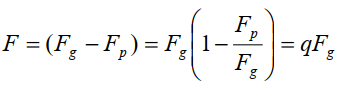Where,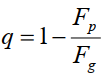is the mass radiation factor. We denote the radiation factor as q such 0 < 1- q << 1.

1.7.4 Oblateness
The degree of flattening of a celestial object, such as a planet, from a true spherical form, largely as a result of rotation is called the oblateness of the celestial object. It is numerically equal to the difference between the equatorial and polar diameter of the object divided by the eccentric diameter.

The rotation of a star produces an equatorial bulge due to centrifugal force; as a result, the stars are often oblate in shape. Neutron stars and black dwarfs which is a result of the cooling of white dwarfs are also oblate due to their rapid spinning after formation, For example, the Earth, Jupiter, Saturn and stars (Archerner, Antares and Altair) have the shape of an oblate spheroid.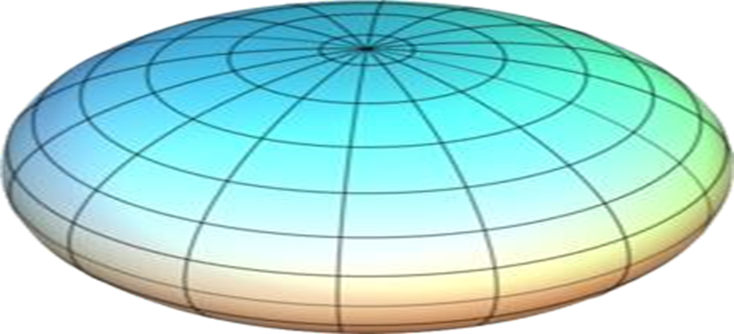Figure 1. 1: The shape of an oblate spheroid

1.7.5 Eccentricity
The orbital eccentricity of an astronomical body is the amount by which its orbit deviates from a perfect circle and is usually denoted by e. Where for e < 1 the orbit is elliptic; for e = 0, the orbit is circle; for e = 1 the orbit is parabolic and for e > 1 the orbit is hyperbolic.

1.7.6 Synodic or Rotating Co-ordinate System

If the system of coordinates is such that the X -Y plane rotates in the positive direction with an angular velocity equal to that of the common velocity of one primary with respect to the other keeping the origin fixed, then the coordinate system is known as synodic co- ordinate system. The synodic co-ordinate system is accelerated, since it is rotating with a velocity.

1.7.7 Equilibrium Point and Stability of Equilibrium Point
A particle is said to be at equilibrium point if it is stationary at that point or it is in a steady state. Mathematically, if a dynamical system is in state of equilibrium it remains in that state as t ® ¥. Precisely, we can say that, a system of ordinary differential equation  = X (x), which possess a point x = xeq Where x = (x1 , x2 ,...,xn and the component of n-vector X = (X1,X2,...,Xn). A Solution x(t) is called the equilibrium point where x(to ) = xeq This point is obtained from equation X(x) = 0, that is, x = 0 is the equilibrium point. A solution x = xeq  or a point ' xeq is said to be stable if for a given e > there exist a d (x) > such that when the disturbances satisfy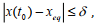then for all t > t0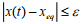. Otherwise, the equilibrium point x = xeq is unstable.

Mathematically for asymptotic stability,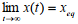1.7.8 Stability of a Linear System

If the system of differential equation x = X (t) can be written in the form x = Ax + f (x) then the linearized system of equation is x = Ax . Where A is a constant matrix and f(x) is a vector function such that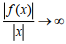for all t ³ t0
The stability condition for t  t0 of the linearized system can be stated as:
i. For complex root of characteristic equation of A, we have
When the characteristic roots all have negative and also when some of the roots are multiple, then the equilibrium point are stable.
When some or all the characteristic roots have positive real part, the equilibrium points are unstable. This is also valid for multiple roots.

ii. For pure imaginary roots, the motion is oscillatory and the solution is stable though not asymptotically stable. If these are multiple roots the solution contains mixed (period and secular) terms and the equilibrium point is unstable.

iii. If the roots are real and all negative, the solution is stable; if, however, any of the roots are positive the point is unstable. These statements are also true for multiple roots.

1.7.9 Binary Star System

A binary star is a star system having two stars orbiting around their common center of mass. Systems of three, four, or even more stars are called multiple star systems. These systems, especially when more distant, often appear to the unaided eye as a single point of light, and are then revealed as double (or more) by other means. Over the last two centuries, researches have suggests that half or more of visible stars are part of multiple star systems (Filippenko, 2007).

In fact, most stars exist in binary systems. The Sun, with its collection of planets, is an exception. Stars in binaries revolve around their common center of mass (which itself moves through space). Examples of binary systems include among many, CM Draconis, Sirius, Achird, Luyten 726-8, Kruger 60, Alpha Centauri AB, Xi Bootis and Gamma Leporis, etc.

1.7.10 Circumbinary Planets

A planet orbiting two stars instead of one is called a circumbinary planet. Because of the short orbits of some binary stars, the only way for planets to form is by forming outside the orbit of the two stars. The system PSR B1620-26, is being orbited by the first confirmed circumbinary extrasolar planet, containing a millisecond pulsar and a white dwarf and is located in the globular cluster M4. In 1993 the existence of the third body was first reported, and was suggested to be a planet based on 5 years of observational data. In 2003 the planet was characterized as being 2.5 times the mass of Jupiter in a low eccentricity orbit with a semi major axis of 23 AU.

1.7.11 Circumbinary Disc

Circumbinary disc that may indicate processes of planet formation have been found around several stars, and are in fact common around binaries with separations less than 3 AU (Trilling et al.  2007). One notable example is in the HD 98800 system, which comprises two pairs of binary stars separated by around 34 AU (Fig 1.2). The binary subsystem HD 98800 B, which consists of two stars of 0.70 and 0.58 solar masses in a highly eccentric orbit with semi major axis 0.983 AU, is surrounded by a complex dust disc that is being warped by the gravitational effects of the mutually-inclined and eccentric stellar orbits (Akeson et al. 2007; Verrier and Evans 2008).

1.7.12 Protoplanetary Disc

A protoplanetary disc is a rotating circumstellar disc of dense gas and dust surrounding a young newly formed star, a T Tauri star, or Herbig Ae/Be star. The protoplanetary disc may also be considered as an accretion disc for the star itself, because gasses or other material may be falling from the inner edge of the disc onto the surface of the star. But this process should not be confused with the accretion process thought to build up the planets themselves. Externally illuminated photo-evaporating protoplanetary discs are called proplyds. Protoplanetary disks have been observed around several young stars in our galaxy. Recent observations by the Hubble Space Telescope have shown proplyds and planetary discs to be forming within the Orion Nebula. Protoplanetary disc are thought to be thin structures, with a typical vertical height much smaller than the radius, and a typical mass much smaller than the Central young star (Armitage, 2011).

1.7.13 Debris Disc

Gas (poor disc) of circumstellar dust have been found around many nearby stars-most of which have ages in the range of ~10 million years (e.g. Beta Pictoris, 51 Ophiuchi) to billions of years (e.g. Tau Ceti). These systems are usually referred to as "debris discs". Given the older ages of these stars, and the short lifetimes of micrometer-sized dust grains around stars due to Poynting Robertson drag, collisions, and radiation pressure (typically hundreds to thousands of years), it is thought that this dust is from the collisions of planetesimals (e.g. asteroids, comets). Hence the debris discs around these examples (e.g. Vega, Alphecca, Fomalhaut, etc.) are probably not truly "protoplanetary", but represent a later stage of disc evolution, where extrasolar analogs of the asteroid belt and Kuiper belt are home to dust-generating collisions between planetesimals.

1.7.14 Asteroid belt

The asteroid belt is the circumstellar disc in the Solar System located roughly between the orbits of the planets Mars and Jupiter. It is occupied by numerous irregularly shaped bodies called asteroids or minor planets. The asteroid belt is also termed the main asteroid belt or main belt to distinguish it from other asteroid populations in the Solar System such as near-Earth asteroids and Trojan asteroids, about half the mass of the belt is contained in the four largest asteroids: Ceres, Vesta, Pallas, and Hygiea as observed by Matt, W. (2015). The total mass of the asteroid belt is approximately 4% that of the Moon, or 22% that of Pluto, and roughly twice that of Pluto's moon Charon (whose diameter is 1200 km).

1.7.15 Asteroid with Disc

Hitherto, rings have been found exclusively around the four giant planets in the Solar System as stated by Tiscarena, (2013). Rings are natural laboratories in which to study dynamical processes analogous to those that take place during the formation of planetary systems and galaxies. Their presence also tells us about the origin and evolution of the body they encircle. Observations of a multichord stellar occultation that revealed the presence of a ring system around Chariklo (10199), which is a Centaur-that is, one of a class of small objects orbiting where also stipulated by Fornasier, et al (2013), primarily between Jupiter and Neptune-with an equivalent radius of 124+9 kilometers. There are two dense rings, with respective widths of about 7 and 3 kilometers, optical depths of 0.4 and 0.06, and orbital radii of 391 and 405 kilometers. The present orientation of the ring is consistent with an edge-on geometry in 2008, which provides a simple explanation for the dimming as explained by Belskaya, (2010) of the Chariklo system between1997 and 2008, and for the gradual disappearance of ice and other absorption features in its spectrum over the same period as forwarded by Guilbert, A. et al. (2009). This implies that the rings are partly composed of water ice. They may be the remnants of a debris disc, possibly confined by embedded, kilometer-sized satellites. Chariklo is the largest known Centaur orbiting in a region between Saturn and Uranus, and has an orbital eccentricity of 0.175 and a semi major axis of 15.8 astronomical units (1 AU is the Earth- Sun distance). It may be a former trans-Neptunian object that has been recently (less than lOMyr ago) scattered by gravitational perturbations from Uranus as indicated by Horner, et al (2004), No clear detection of Chariklo's rotation has been made so far. Its surface is very dark with a geometric albedo of 0.035 ± 0.011 as pointed out by Fornasier, et al (2013)., and it is subject to long-term spectral and photometric variabilities (Belskaya, 2010). Other evidence supporting the interpretation of a flat circular ring system around Chariklo are given by Braga- Ribas et al, (2014).

1.7.16 CM Draconis

CM Draconis (GJ 630.1A) is an eclipsing binary system approximately 47 light-years away (Van, 1995) in the constellation of Draco (the Dragon). The system consists of two nearly identical red dwarf stars located in the constellation Draco. The two stars orbit each other with a period of 1.27 days with a separation of 2.7 million kilometers (0.018 AU). Along with two stars in the triple system KOI 126, the stars in CM Draconis are the lightest stars with precisely measured masses and radii. Consequently, the system plays an important role in testing stellar structure models for very low mass stars (Morales et al. 2009). These comparisons find that models under predict the stellar radii by approximately 5%. This is attributed to consequences of the stars' strong magnetic activity. According to the system's entry in the Combined General catalogue of variable stars, at least one of the components is a flare star and at least one is a BY Draconis variable. The white dwarf star GJ 630.1B, located 25.7 arc seconds away shares the same proper motion as the CM Draconis stars and is thus a true companion star of the system (Deeg et al, 2008), Given the system's distance of 47 light years, this corresponds to a separation of at least 370 astronomical units between CM Draconis and GJ 630.1B (Gliese et al, 1991).

1.7.17 HD 98800

HD 98800, also catalogued as TV Crateris (TV Crt), is a quadruple star system approximately 150 light-years away in the constellation of Crater (the cup). The system is located within the TW Hydrae association. The system consists of "HD 98800 A" and "HD 98800 B" each of which contains two stars.

In 2007, a debris disc was discovered orbiting "HD 98800 B" consisting of two rings which indicates there may be an extrasolar planet orbiting within a distance of 1.5 to 2 astronomical units. The system consists of four T Tauri stars that are located within the TW Hydrae association. Within the system, there are two separate systems of binary stars, each system (separately catalogued as "HD 98800 A" and "HD 98800B") consists of two stars. Although the four stars are gravitationally bound, the distance separating the two binary pairs is about 50 astronomical units (somewhat more than the average distance between the Sun and Pluto). Not much is known about each individual star, except that they are basically solar twin stars.